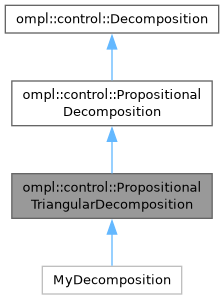ompl::control::PropositionalTriangularDecomposition Class Reference

A PropositionalTriangularDecomposition is a triangulation that ignores obstacles and respects propositional regions of interest. Practically speaking, it is both a TriangularDecomposition and a PropositionalDecomposition, but it is implemented without using multiple inheritance. More...

#include <ompl/extensions/triangle/PropositionalTriangularDecomposition.h>

Inheritance diagram for ompl::control::PropositionalTriangularDecomposition:[legend]

## Public Types

using Polygon = TriangularDecomposition::Polygon

using Vertex = TriangularDecomposition::Vertex

## Public Member Functions

PropositionalTriangularDecomposition (const base::RealVectorBounds &bounds, const std::vector< Polygon > &holes=std::vector< Polygon >(), const std::vector< Polygon > &props=std::vector< Polygon >())
Creates a PropositionalTriangularDecomposition over the given bounds, which must be 2-dimensional. The underlying mesh will be a conforming Delaunay triangulation. The triangulation will ignore any obstacles, given as a list of polygons. The triangulation will respect the boundaries of any propositional regions of interest, given as a list of polygons.

int getNumProps () const override
Returns the number of propositions in this propositional decomposition.

World worldAtRegion (int triID) override
Returns the World corresponding to a given region.

void setup ()

const std::vector< Polygon > & getHoles () const

const std::vector< Polygon > & getPropositions () const

void print (std::ostream &out) constPublic Member Functions inherited from ompl::control::PropositionalDecomposition
PropositionalDecomposition (const DecompositionPtr &decomp)
Creates a propositional decomposition wrapped around a given decomposition with a given number of propositions.

~PropositionalDecomposition () override
Clears all memory belonging to this propositional decomposition.

int getNumRegions () const override
Returns the number of regions in this propositional decomposition's underlying decomposition.

double getRegionVolume (int rid) override
Returns the volume of a given region.

int locateRegion (const base::State *s) const override
Returns the region of the underlying decomposition that contains a given State.

void project (const base::State *s, std::vector< double > &coord) const override
Project a given State to a set of coordinates in R^k, where k is the dimension of this Decomposition.

void getNeighbors (int rid, std::vector< int > &neighbors) const override
Stores a given region's neighbors into a given vector.

void sampleFromRegion (int rid, RNG &rng, std::vector< double > &coord) const override
Samples a projected coordinate from a given region.

void sampleFullState (const base::StateSamplerPtr &sampler, const std::vector< double > &coord, base::State *s) const override
Samples a State using a projected coordinate and a StateSampler.Public Member Functions inherited from ompl::control::Decomposition
Decomposition (int dim, const base::RealVectorBounds &b)
Constructor. Creates a Decomposition with a given dimension and a given set of bounds. Accepts as an optional argument a given number of regions.

virtual int getDimension () const
Returns the dimension of this Decomposition.

virtual const base::RealVectorBoundsgetBounds () const
Returns the bounds of this Decomposition.

## Protected Attributes

TriangularDecompositiontriDecomp_Protected Attributes inherited from ompl::control::PropositionalDecomposition
DecompositionPtr decomp_Protected Attributes inherited from ompl::control::Decomposition
int dimension_

base::RealVectorBounds bounds_

## Detailed Description

A PropositionalTriangularDecomposition is a triangulation that ignores obstacles and respects propositional regions of interest. Practically speaking, it is both a TriangularDecomposition and a PropositionalDecomposition, but it is implemented without using multiple inheritance.

Definition at line 58 of file PropositionalTriangularDecomposition.h.

The documentation for this class was generated from the following files: